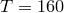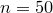Want to share your content on R-bloggers? click here if you have a blog, or here if you don't.

A common model for counts data is the Poisson. There are cases however that we only record positive counts, ie there is a truncation of 0. This is the truncated Poisson model.

To study this model we only need the total counts and the sample size. This comes from the sufficient statistic principle as the likelihood is,

where. Let’s setand.

```sum.x=160
n=50
library(maxLik)

loglik <- function(theta, n, sum.x) {
- n* log(exp(theta) - 1) + sum.x * log(theta)
}```.

```gradlik <- function(theta, n, sum.x){
(n*exp(-2*theta))/(1-exp(-theta))^2
+(n*exp(-theta))/(1-exp(-theta))-sum.x/(theta^2)
}```

The second derivative (the hessian) is.

```hesslik <- function(theta, n, sum.x) {
-((2*n*exp(-2*theta))/(1-exp(-theta))^2)
-(n*exp(-theta))/(1-exp(-theta))
-exp(-theta)*((2*exp(-2*theta))/(1-exp(-theta))^3
+exp(-theta)/(1-exp(-theta))^2)*n+(2*sum.x)/theta^3}```

Now, we simply call the s/t function of maxLik package to fit the model.

```a <- maxLik(loglik, start=3.2,method="Newton-Raphson",
n=n,sum.x=sum.x, print.level=2)
# ----- Initial parameters: -----
# fcn value: 28.18494
# [1,]       3.2        -2.124718    1
# Condition number of the (active) hessian: 1
# -----Iteration 1 -----
# -----Iteration 2 -----
# -----Iteration 3 -----
# --------------
# gradient close to zero. May be a solution
# 3  iterations
# estimate: 3.048175
# Function value: 28.34853

```

There is an nice & handy function I found somewhere in the net (sorry I don’t remember the author;() to plot the likelihood of the truncated Poisson.

```plot.logL = function(Left, Right, Step){
grid.x = seq(Left, Right, by = Step)
plot(grid.x, loglik(grid.x, n, sum.x), type = "l", ,col="red",
xlab = "theta", ylab = "logL",
main = "Log likelihood for truncated Poisson")
}
plot.logL(2, 4, 0.00001);grid()```